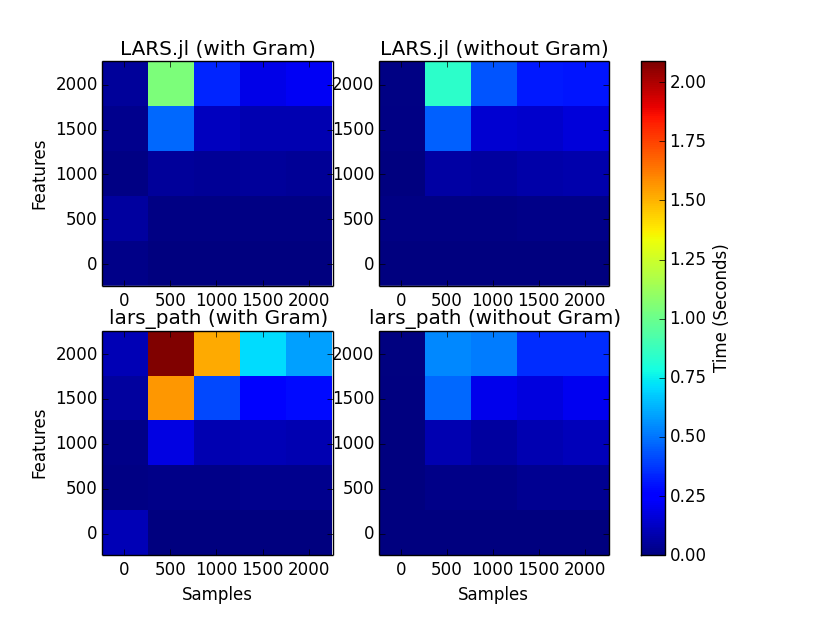16

1

6

1

# Least angle regression

## Introduction

Least angle regression is a variable selection/shrinkage procedure for high-dimensional data. It is also an algorithm for efficiently finding all knots in the solution path for the aforementioned this regression procedure, as well as for lasso (L1-regularized) linear regression. Fitting the entire solution path is useful for selecting the optimal value of the shrinkage parameter λ for a given dataset, and for the lasso covariance test, which provides the significance of each variable addition along the lasso path.

## Usage

LARS solution paths are provided by the `lars` function:

``````lars(X, y; method=:lasso, intercept=true, standardize=true, lambda2=0.0,
use_gram=true, maxiter=typemax(Int), lambda_min=0.0, verbose=false)
``````

`X` is the design matrix and `y` is the dependent variable. The optional parameters are:

`method` - either `:lasso` or `:lars`.

`intercept` - whether to fit an intercept in the model. The intercept is always unpenalized.

`standardize` - whether to standardize the predictor matrix. In contrast to linear regression, this affects the algorithm's results. The returned coefficients are always unstandardized.

`lambda2` - the elastic net ridge penalty. Zero for pure lasso. Note that the returned coefficients are the "naive" elastic net coefficients. They can be adjusted as recommended by Zhou and Hastie (2005) by scaling by `1 + lambda2`.

`use_gram` - whether to use a precomputed Gram matrix in computation.

`maxiter` - maximum number of iterations of the algorithm. If this is exceeded, an incomplete path is returned. `lambda_min` - value of λ at which the algorithm should stop.

`verbose` - if true, prints information at each step.

The `covtest` function computes the lasso covariance test based on a LARS path:

`covtest(path, X, y; errorvar)`

`path` is the output of the LARS function above, and `X` and `y` are the independent and dependent variables used in fitting the path. If specified, `errorvar` is the variance of the error. If not specified, the error variance is computed based on the least squares fit of the full model.

## Notes

The output of `covtest` has minor discrepancies with that of the covTest package. This is because the covTest package does not take into account the intercept in the least squares model fit when computing the error variance, which I believe is incorrect. I have emailed the authors but have yet to receive a response.

## BenchmarksLARS.jl is substantially faster than scikit-learn for cases where the number of samples exceeds the number of features, particularly when using a Gram matrix. For cases where the number of features greatly exceeds the number of samples, scikit-learn is still occasionally faster. I am still tracking down the cause.

GLMNet fits the lasso solution path using coordinate descent and supports fitting L1-regularized generalized linear models.

## Credits

This package is written and maintained by Simon Kornblith simon@simonster.com.

The `lars` function is derived from code from scikit-learn written by:

03/21/2014

14 days ago

28 commits